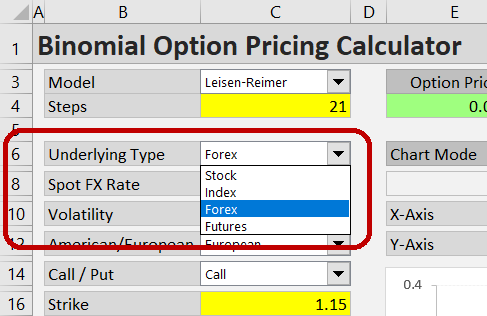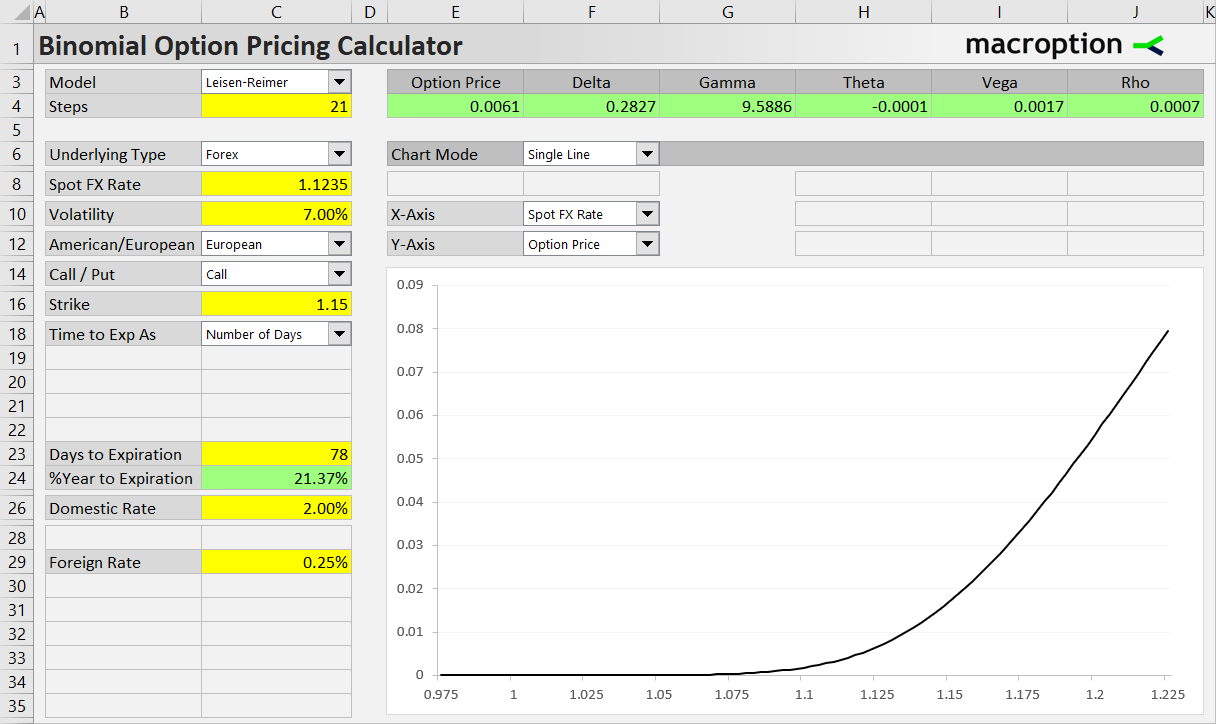# Currency Options

This page explains how to price forex options in the Binomial Option Pricing Calculator and discusses some of their unique features.

## Entering Inputs

The calculator can work with stock, index, currency or futures options. This is selected in the Underlying Type dropdown box in cell C6. For currency options, select “Forex”.Most of the inputs work generally the same as with the other underlying types, but there are a few small differences due to specific characteristics of FX options.

## Two Interest Rates

The most important one, which differentiates currency options from all the other option types, is that their prices are affected by two interest rates instead of one, because two currencies are involved:

• The “domestic” interest rate is the rate on the currency in which the option is denominated – the currency in which you pay the option premium.
• The “foreign” interest rate is the rate on the currency which is the option’s underlying – the currency you are buying when exercising a call, or selling when exercising a put.

For instance, for an option on the EUR/USD exchange rate which trades in dollars and you get euros for dollars when exercising a call, USD rate is the domestic rate and EUR rate is the foreign rate.

Note that like the “American” and “European” option types, the terms “domestic” and “foreign” don’t necessarily refer to the location of the trading, but to the interest rate on funding (domestic) and the yield on the option’s underlying currency (foreign). Instead of domestic and foreign rate, we might as well call them “funding rate” and “yield”.

## Foreign Rate Is Like Dividend Yield

In fact, the role of the “foreign” interest rate in the option pricing models (not only binomial models but also Black-Scholes / Garman-Kohlhagen, for instance) is exactly the same as the role of dividend yield for stock or index options. Mathematically they are the same thing – the rate you earn while holding the underlying security, but not when holding a call option. Therefore the higher it is, the lower value calls have (and the higher value puts have), other factors being equal.

## Entering Interest Rates in the Calculator

In the Binomial Option Pricing Calculator, enter the domestic rate in the yellow cell C26 – same as interest rate for other underlying types.

Enter the foreign rate in the yellow cell C29, which is the same cell where dividend yield is entered for stock and index options, for the reason explained above.

Either rate can be positive or negative or zero (the calculator accepts any rate from -50% to +100%).

## Example

Let’s price a call option on the EUR/USD exchange rate, which (EUR/USD spot) is currently at 1.1235. The option’s strike price (or strike rate) is 1.1500. USD interest rate (domestic or funding rate) is 2.00%, EUR rate (foreign rate or yield) is 0.25%. The option is European type, expires in 78 days, and we expect volatility of 7%.

The correct inputs are shown in the screenshot below.The resulting option price, shown in the green cell E4, is 0.0061, or 61 pips. You can also see the Greeks next to it.

## Underlying Rate and Strike Units

Another characteristic of forex is than many rates, especially the major pairs, are nominally very small numbers close to 1 or even below. Furthermore, option prices are often only small fractions of underlying price. As a result, we are working with very small numbers, where interesting things only happen at the fourth or fifth digit after the decimal point. For instance, in our example, theta is showing -0.0001, which can be 0.5 to 1.5 pips per day – a big difference. When you are working with small rates, there are two ways to see more precise option prices and Greeks.

One is to change the number format of the green cells E4-J4 to display more decimal places. The macros don’t touch it and it is safe to change. Note the calculator rounds results to 10 decimal places by default – you can change this setting in Preferences.

A better way is to work with bigger numbers from the start. Instead of the actual spot rate like 1.1235, multiply it by 100 and enter the underlying FX rate as 112.35. You can even enter it in pips as 11235 – in such case the resulting option prices and Greeks will be directly in pips. Only thing to keep in mind is to always enter the underlying rate (cell C8) and strike (cell C16) in the same units: either 1.1235 and 1.15, or 112.35 and 115, or 11235 and 11500.

## Other Underlying Types

Besides the special features discussed above, pricing currency options works the same as other types of options – some of them have their own special features. For instructions and examples for the other underlying types, see: